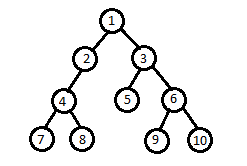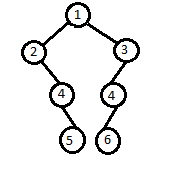Problem title
Difficulty
Avg time to solve

Is it a Circular Linked List?
Easy
15 mins
Maximum Area Square
Moderate
10 mins
Sum Of Three Stacks
Easy
10 mins
Print All The Nodes At Distance K From The Given Node
Moderate
20 mins
Reversing a Queue
Easy
10 mins
Minimum Travel Cost
Moderate
40 mins
Queen's Palace
Moderate
25 mins
Count smaller people on right side
Easy
10 mins
Find Odd Occurrence Element
Easy
10 mins
Longest Repeating Subsequence
Moderate
15 mins4

# Leftmost & Rightmost Nodes of Binary Tree

Difficulty: EASY
Avg. time to solve
20 min
Success Rate
80%

Problem Statement

#### Given a Binary Tree of 'N' number of total nodes, return the sequence of values of the leftmost and rightmost node at each level.

##### For example:
``````For the given binary tree:
````````````Output: 1 2 3 4 6 7 10

Explanation: The leftmost and rightmost node respectively of each level are
Level 0: 1(only one node is present at 0th level)
Level 1: 2 3
Level 2: 4 6
Level 3: 7 10
``````
##### Input Format:
``````The only line of input contains tree elements in the level order form. The input consists of values of nodes separated by a single space in a single line. In case a node is null, we take -1 in its place.

For example, the input for the tree depicted in the below image would be :
````````````1
2 3
4 -1 5 6
-1 7 -1 -1 -1 -1
-1 -1
``````

#### Explanation :

``````Level 1 :
The root node of the tree is 1

Level 2 :
Left child of 1 = 2
Right child of 1 = 3

Level 3 :
Left child of 2 = 4
Right child of 2 = null (-1)
Left child of 3 = 5
Right child of 3 = 6

Level 4 :
Left child of 4 = null (-1)
Right child of 4 = 7
Left child of 5 = null (-1)
Right child of 5 = null (-1)
Left child of 6 = null (-1)
Right child of 6 = null (-1)

Level 5 :
Left child of 7 = null (-1)
Right child of 7 = null (-1)

The first not-null node (of the previous level) is treated as the parent of the first two nodes of the current level. The second not-null node (of the previous level) is treated as the parent node for the next two nodes of the current level and so on.

The input ends when all nodes at the last level are null (-1).
``````
##### Note :
``````The above format was just to provide clarity on how the input is formed for a given tree.
The sequence will be put together in a single line separated by a single space. Hence, for the above-depicted tree, the input will be given as:

1 2 3 4 -1 5 6 -1 7 -1 -1 -1 -1 -1 -1
``````
##### Output Format:
``````For each test case, print a single line containing space-separated integers denoting a sequence of integers written progressively from top to bottom of the tree such that for each level, left most data is followed by the rightmost one.

The output of each test case will be printed in a separate line.
``````
##### Note:
``````You do not need to print anything; it has already been taken care of. Just implement the given function.
``````
##### Constraints:
``````0 <= N <= 10 ^ 5

Time Limit: 1 sec.
``````
##### Sample Input 1:
``````1 2 3 -1 -1 -1 -1
``````
##### Sample Output 1:
``````1 2 3
``````
##### Sample Input 2:
``````1 2 3 -1 4 4 -1 -1 5 6 -1 -1 -1 -1 -1
``````
##### Sample Output 2:
``````1 2 3 4 4 5 6
``````
##### Explanation to Sample Input 2:
``````The input binary tree will be represented as
````````````From the above representation, the leftmost and rightmost node respectively of each level are:
Level 0: 1 (only one node is present at 0th level)
Level 1: 2 3
Level 2: 4 4
Level 3: 5 6

Note: Do not consider the vertical distance of nodes from the root node to find the leftmost and rightmost nodes. As in this example, at level 3(0 based indexing) the node with value 5 will have a vertical distance of +1 from the root node and the node with value 6 will have a vertical distance of -1 from the root node.
``````Console